Download Presentation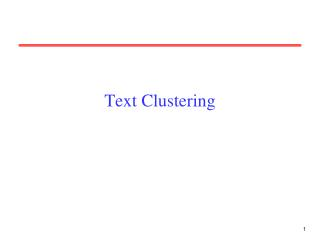Text Clustering

# Text Clustering - PowerPoint PPT Presentation

Text Clustering. Clustering. Partition unlabeled examples into disjoint subsets of clusters , such that: Examples within a cluster are very similar Examples in different clusters are very different Discover new categories in an unsupervised manner (no sample category labels provided). .I am the owner, or an agent authorized to act on behalf of the owner, of the copyrighted work described.
Download Presentation## Text Clustering

An Image/Link below is provided (as is) to download presentation

Download Policy: Content on the Website is provided to you AS IS for your information and personal use and may not be sold / licensed / shared on other websites without getting consent from its author.While downloading, if for some reason you are not able to download a presentation, the publisher may have deleted the file from their server.

- - - - - - - - - - - - - - - - - - - - - - - - - - E N D - - - - - - - - - - - - - - - - - - - - - - - - - -
Presentation Transcript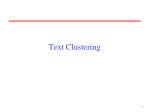### Text Clustering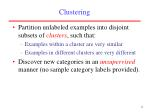Clustering
• Partition unlabeled examples into disjoint subsets of clusters, such that:
• Examples within a cluster are very similar
• Examples in different clusters are very different
• Discover new categories in an unsupervised manner (no sample category labels provided).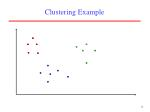.

.

.

.

.

.

.

.

.

.

.

.

.

.

.

.

.

.

.

.

.

.

.

.

.

.

.

.

.

.

.

Clustering Example

.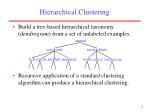animal

vertebrate

invertebrate

fish reptile amphib. mammal worm insect crustacean

Hierarchical Clustering
• Build a tree-based hierarchical taxonomy (dendrogram) from a set of unlabeled examples.
• Recursive application of a standard clustering algorithm can produce a hierarchical clustering.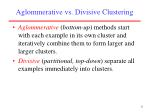Aglommerative vs. Divisive Clustering
• Aglommerative (bottom-up) methods start with each example in its own cluster and iteratively combine them to form larger and larger clusters.
• Divisive (partitional, top-down) separate all examples immediately into clusters.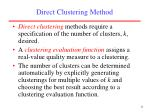Direct Clustering Method
• Direct clustering methods require a specification of the number of clusters, k, desired.
• A clustering evaluationfunctionassigns a real-value quality measure to a clustering.
• The number of clusters can be determined automatically by explicitly generating clusterings for multiple values of k and choosing the best result according to a clustering evaluation function.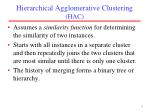Hierarchical Agglomerative Clustering (HAC)
• Assumes a similarity function for determining the similarity of two instances.
• Starts with all instances in a separate cluster and then repeatedly joins the two clusters that are most similar until there is only one cluster.
• The history of merging forms a binary tree or hierarchy.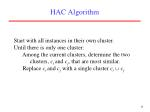HAC Algorithm

Start with all instances in their own cluster.

Until there is only one cluster:

Among the current clusters, determine the two

clusters, ci and cj, that are most similar.

Replace ci and cj with a single cluster ci cj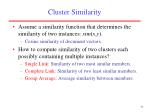Cluster Similarity
• Assume a similarity function that determines the similarity of two instances: sim(x,y).
• Cosine similarity of document vectors.
• How to compute similarity of two clusters each possibly containing multiple instances?
• Single Link: Similarity of two most similar members.
• Complete Link: Similarity of two least similar members.
• Group Average: Average similarity between members.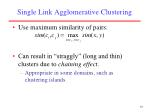Single Link Agglomerative Clustering
• Use maximum similarity of pairs:
• Can result in “straggly” (long and thin) clusters due to chaining effect.
• Appropriate in some domains, such as clustering islands.Complete Link Agglomerative Clustering
• Use minimum similarity of pairs:
• Makes more “tight,” spherical clusters that are typically preferable.Computational Complexity
• In the first iteration, all HAC methods need to compute similarity of all pairs of n individual instances which is O(n2).
• In each of the subsequent n2 merging iterations, it must compute the distance between the most recently created cluster and all other existing clusters.
• In order to maintain an overall O(n2) performance, computing similarity to each other cluster must be done in constant time.Computing Cluster Similarity
• After merging ci and cj, the similarity of the resulting cluster to any other cluster, ck, can be computed by:Group Average Agglomerative Clustering
• Use average similarity across all pairs within the merged cluster to measure the similarity of two clusters.
• Compromise between single and complete link.
• Averaged across all ordered pairs in the merged cluster instead of unordered pairs between the two clusters (to encourage tighter final clusters).Computing Group Average Similarity
• Assume cosine similarity and normalized vectors with unit length.
• Always maintain sum of vectors in each cluster.
• Compute similarity of clusters in constant time:Non-Hierarchical Clustering
• Typically must provide the number of desired clusters, k.
• Randomly choose k instances as seeds, one per cluster.
• Form initial clusters based on these seeds.
• Iterate, repeatedly reallocating instances to different clusters to improve the overall clustering.
• Stop when clustering converges or after a fixed number of iterations.K-Means
• Assumes instances are real-valued vectors.
• Clusters based on centroids, center of gravity, or mean of points in a cluster, c:
• Reassignment of instances to clusters is based on distance to the current cluster centroids.Distance Metrics
• Euclidian distance (L2 norm):
• L1 norm:
• Cosine Similarity (transform to a distance by subtracting from 1):K-Means Algorithm

Let d be the distance measure between instances.

Select k random instances {s1, s2,… sk} as seeds.

Until clustering converges or other stopping criterion:

For each instance xi:

Assign xi to the cluster cjsuch that d(xi, sj) is minimal.

(Update the seeds to the centroid of each cluster)

For each cluster cj

sj = (cj)Pick seeds

Reassign clusters

Compute centroids

Reasssign clusters

x

x

x

Compute centroids

x

x

x

K Means Example(K=2)

Reassign clusters

Converged!Time Complexity
• Assume computing distance between two instances is O(m) where m is the dimensionality of the vectors.
• Reassigning clusters: O(kn) distance computations, or O(knm).
• Computing centroids: Each instance vector gets added once to some centroid: O(nm).
• Assume these two steps are each done once for I iterations: O(Iknm).
• Linear in all relevant factors, assuming a fixed number of iterations, more efficient than O(n2) HAC.K-Means Objective
• The objective of k-means is to minimize the total sum of the squared distance of every point to its corresponding cluster centroid.
• Finding the global optimum is NP-hard.
• The k-means algorithm is guaranteed to converge a local optimum.Seed Choice
• Results can vary based on random seed selection.
• Some seeds can result in poor convergence rate, or convergence to sub-optimal clusterings.
• Select good seeds using a heuristic or the results of another method.Buckshot Algorithm
• Combines HAC and K-Means clustering.
• First randomly take a sample of instances of size n
• Run group-average HAC on this sample, which takes only O(n) time.
• Use the results of HAC as initial seeds for K-means.
• Overall algorithm is O(n) and avoids problems of bad seed selection.Text Clustering
• HAC and K-Means have been applied to text in a straightforward way.
• Typically use normalized, TF/IDF-weighted vectors and cosine similarity.
• Optimize computations for sparse vectors.
• Applications:
• During retrieval, add other documents in the same cluster as the initial retrieved documents to improve recall.
• Clustering of results of retrieval to present more organized results to the user (à la Northernlight folders).
• Automated production of hierarchical taxonomies of documents for browsing purposes (à la Yahoo & DMOZ).Soft Clustering
• Clustering typically assumes that each instance is given a “hard” assignment to exactly one cluster.
• Does not allow uncertainty in class membership or for an instance to belong to more than one cluster.
• Soft clustering gives probabilities that an instance belongs to each of a set of clusters.
• Each instance is assigned a probability distribution across a set of discovered categories (probabilities of all categories must sum to 1).Expectation Maximumization (EM)
• Probabilistic method for soft clustering.
• Direct method that assumes k clusters:{c1, c2,… ck}
• Soft version of k-means.
• Assumes a probabilistic model of categories that allows computing P(ci | E)for each category, ci, for a given example, E.
• For text, typically assume a naïve-Bayes category model.
• Parameters  = {P(ci), P(wj | ci): i{1,…k}, j {1,…,|V|}}EM Algorithm
• Iterative method for learning probabilistic categorization model from unsupervised data.
• Initially assume random assignment of examples to categories.
• Learn an initial probabilistic model by estimating model parameters  from this randomly labeled data.
• Iterate following two steps until convergence:
• Expectation (E-step): Compute P(ci | E)for each example given the current model, and probabilistically re-label the examples based on these posterior probability estimates.
• Maximization (M-step): Re-estimate the model parameters, , from the probabilistically re-labeled data.+

+

+

+

+

EM

Initialize:

Assign random probabilistic labels to unlabeled data

Unlabeled ExamplesProb. Learner

+

+

+

+

+

EM

Initialize:

Give soft-labeled training data to a probabilistic learnerProb. Learner

Prob.

Classifier

+

+

+

+

+

EM

Initialize:

Produce a probabilistic classifierProb. Learner

Prob.

Classifier

+

+

+

+

+

EM

E Step:

Relabel unlabled data using the trained classifierProb. Learner

Prob.

Classifier

+

+

+

+

+

EM

M step:

Retrain classifier on relabeled data

Continue EM iterations until probabilistic labels on unlabeled data converge.Learning from Probabilistically Labeled Data
• Instead of training data labeled with “hard” category labels, training data is labeled with “soft” probabilistic category labels.
• When estimating model parameters  from training data, weight counts by the corresponding probability of the given category label.
• For example, if P(c1 | E) = 0.8 and P(c2 | E) = 0.2, each word wj in E contributes only 0.8 towards the counts n1 and n1j, and 0.2 towards the counts n2 and n2j .Naïve Bayes EM

Randomly assign examples probabilistic category labels.

Use standard naïve-Bayes training to learn a probabilistic model

with parameters  from the labeled data.

Until convergence or until maximum number of iterations reached:

E-Step: Use the naïve Bayes model to compute P(ci | E) for

each category and example, and re-label each example

using these probability values as soft category labels.

M-Step: Use standard naïve-Bayes training to re-estimate the

parameters  using these new probabilistic category labels.Semi-Supervised Learning
• For supervised categorization, generating labeled training data is expensive.
• Idea: Use unlabeled data to aid supervised categorization.
• Use EM in a semi-supervised mode by training EM on both labeled and unlabeled data.
• Train initial probabilistic model on user-labeled subset of data instead of randomly labeled unsupervised data.
• Labels of user-labeled examples are “frozen” and never relabeled during EM iterations.
• Labels of unsupervised data are constantly probabilistically relabeled by EM.Training Examples

+

-

-

+

+

Prob. Learner

Prob.

Classifier

+

+

+

+

+

Semi-Supervised EM

Unlabeled ExamplesTraining Examples

+

-

-

+

+

Prob. Learner

Prob.

Classifier

+

+

+

+

+

Semi-Supervised EMTraining Examples

+

-

-

+

+

Prob. Learner

Prob.

Classifier

+

+

+

+

+

Semi-Supervised EMTraining Examples

+

-

-

+

+

Prob. Learner

Prob.

Classifier

+

+

+

+

+

Semi-Supervised EM

Unlabeled ExamplesTraining Examples

+

-

-

+

+

Prob. Learner

Prob.

Classifier

+

+

+

+

+

Semi-Supervised EM

Continue retraining iterations until probabilistic labels on unlabeled data converge.Semi-Supervised EM Results
• Experiments on assigning messages from 20 Usenet newsgroups their proper newsgroup label.
• With very few labeled examples (2 examples per class), semi-supervised EM significantly improved predictive accuracy:
• 27% with 40 labeled messages only.
• 43% with 40 labeled + 10,000 unlabeled messages.
• With more labeled examples, semi-supervision can actually decrease accuracy, but refinements to standard EM can help prevent this.
• Must weight labeled data appropriately more than unlabeled data.
• For semi-supervised EM to work, the “natural clustering of data” must be consistent with the desired categories
• Failed when applied to English POS tagging (Merialdo, 1994)Semi-Supervised EM Example
• Assume “Catholic” is present in both of the labeled documents for soc.religion.christian, but “Baptist” occurs in none of the labeled data for this class.
• From labeled data, we learn that “Catholic” is highly indicative of the “Christian” category.
• When labeling unsupervised data, we label several documents with “Catholic” and “Baptist” correctly with the “Christian” category.
• When retraining, we learn that “Baptist” is also indicative of a “Christian” document.
• Final learned model is able to correctly assign documents containing only “Baptist” to “Christian”.Issues in Clustering
• How to evaluate clustering?
• Internal:
• Tightness and separation of clusters (e.g. k-means objective)
• Fit of probabilistic model to data
• External
• Compare to known class labels on benchmark data
• Improving search to converge faster and avoid local minima.
• Overlapping clustering.Conclusions
• Unsupervised learning induces categories from unlabeled data.
• There are a variety of approaches, including:
• HAC
• k-means
• EM
• Semi-supervised learning uses both labeled and unlabeled data to improve results.